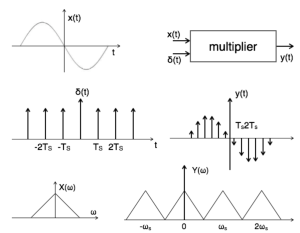# Sampling Theorem

SAMPLING:   A continuous time signal can be represented in its samples and can be recovered back when sampling frequency fs is greater than or equal to the twice the highest frequency (fm) component of message signal. i. e  fs 2fm.

Consider a continuous time signal x(t). The spectrum of x(t) is a band limited to fm Hz. Sampling of input signal x(t) can be obtained by multiplying x(t) with an impulse train δ(t) of period Ts. The output of multiplier is a discrete signal called sampled signal which is represented with y(t) in the following diagrams:ALIASING:

Different signals to become indistinguishable (or aliases of one another) when sampled is called Aliasing. Aliasing occurs when a system is measured at an insufficient sampling rate.Reference:

http://www.tutorialspoint.com/signals_and_systems/signals_sampling_theorem.htm